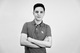1 comment

• Official commentGurobi Staff

Dear Ray,

I'd use two types of general constraints, namely model.addGenConstrAbs() and model.addGenConstrIndicator(), along with a few auxiliary variables. In Python, this would look as follows:

import gurobipy as gpfrom gurobipy import GRBK = 5BigM = 15model = gp.Model("myModel")x = model.addVar(lb=-10, ub=10, vtype=GRB.INTEGER, name="x")y = model.addVar(vtype=GRB.INTEGER, name="y")z = model.addVar(vtype=GRB.INTEGER, name="z")temp = model.addVar(lb=-GRB.INFINITY, name="temp")abs_temp = model.addVar(name="abs_temp")flag = model.addVar(vtype=GRB.BINARY, name="flag")model.addConstr(temp == x - K, name="diff")model.addGenConstrAbs(abs_temp, temp, name="abs_diff")model.addConstr(abs_temp >= 1 - flag, name="aux_1")model.addConstr(abs_temp <= BigM*(1-flag), name="aux_2")model.addGenConstrIndicator(flag, True, y == z, name="indicator")model.setObjective(x, GRB.MAXIMIZE)

Remark 1: For illustrative purposes, I chose an arbitrary objective function and constant K.

Remark 2: The binary variable flag is equal to 1 if and only if abs_temp is equal to zero (i.e. it's equal to zero if abs_temp > 0).

Remark 3: I bounded the general integer variable x, so that I could compute the minimum value for BigM. In fact, it's always a good idea to bound any general integer variable as much as possible. Therefore, it'd be wise to also bound y and z.